Homework Help Question & Answers

# Question 28 2.5 pts Given the acid equilibrium constants (K), which acid will be the strongest...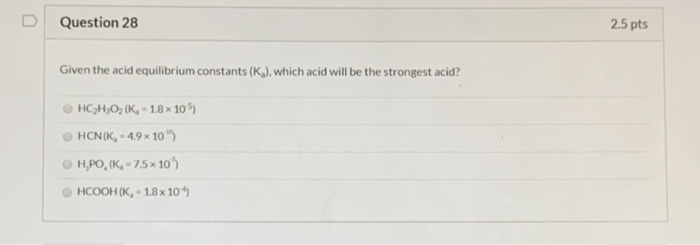Question 28 2.5 pts Given the acid equilibrium constants (K), which acid will be the strongest acid? HC2H302 (K, - 18x105) © HCN (K, 4.9 x 100 H,PO (K, -7.5x 10) HCOOH (K, - 18x104)

#### Homework Answers

Answer #1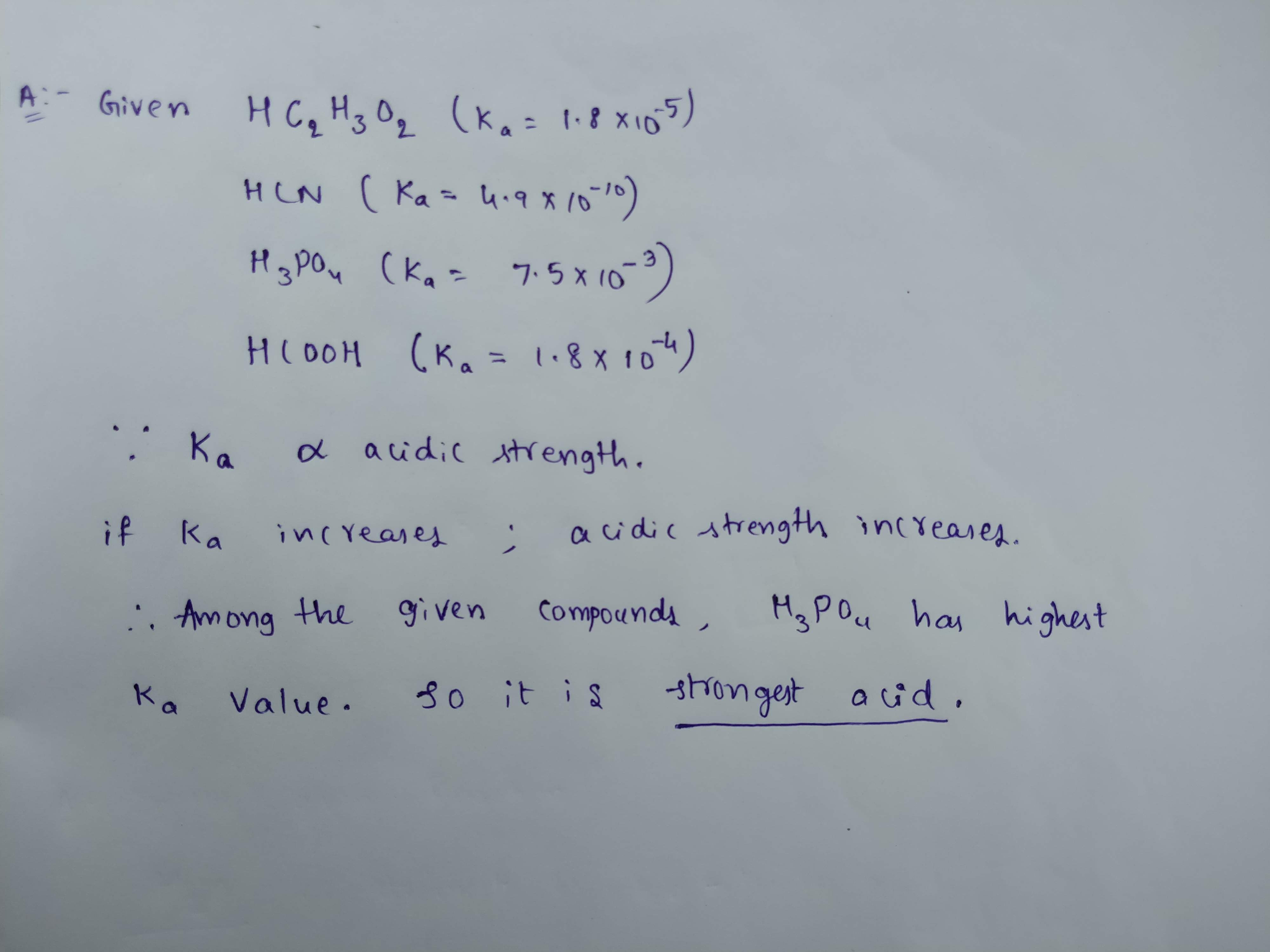Know the answer?
Your Answer:

#### Post as a guest

Your Name:

What's your source?

#### Earn Coin

Coins can be redeemed for fabulous gifts.

Not the answer you're looking for? Ask your own homework help question. Our experts will answer your question WITHIN MINUTES for Free.
Similar Homework Help Questions
• ### QUESTION 12 Based on the acid dissociation constants (K) given, which of these acids is the...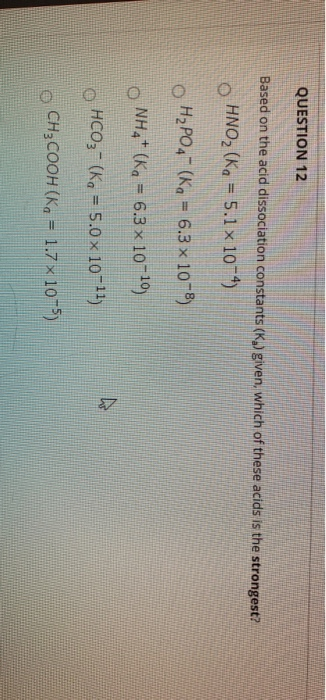QUESTION 12 Based on the acid dissociation constants (K) given, which of these acids is the strongest? HNO2 (Ka = 5.1 x 10-4) H2PO4- (Kg = 6.3 x 10-8) O NH4+ (Kq = 6.3 x 10-10) s HCO3- (Kg = 5.0 x 10-11) CH3COOH (Kg = 1.7 x 1075)

• ### Q 21: Which of the following is the strongest acid? A) HF (K, for HF is...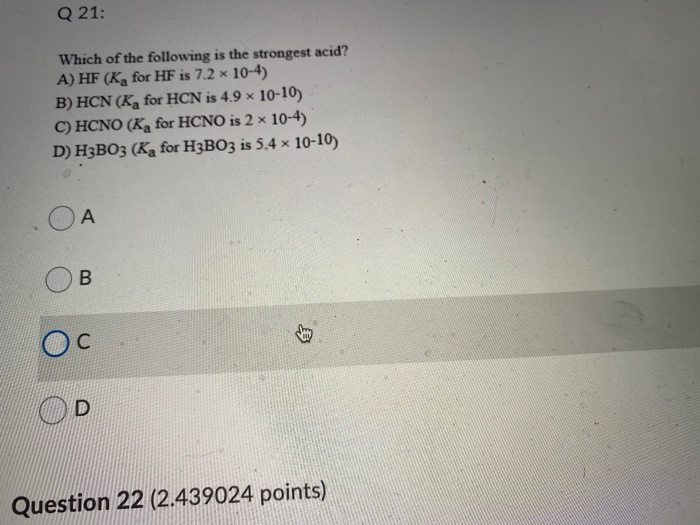Q 21: Which of the following is the strongest acid? A) HF (K, for HF is 7.2 x 10-4) B) HCN (K for HCN is 4.9 x 10-10) C) HCNO (K for HCNO is 2 x 10-4) D) H3B03 (K, for H3B03 is 5.4 x 10-10) OA B с D Question 22 (2.439024 points) We were unable to transcribe this imageQuestion 23 (2.439024 points) Q 23: Which of the following is the weakest acid? A) HF (K, for HF is...

• ### 12. Consider the acid dissociation constants () shown below. Which of the following is the strongest...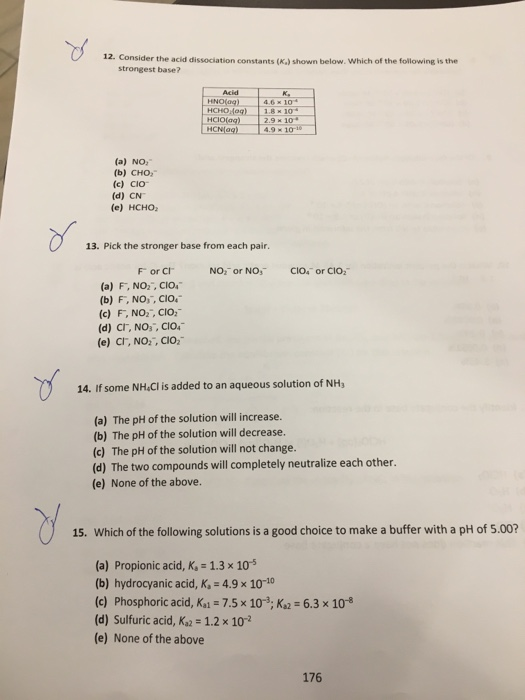12. Consider the acid dissociation constants () shown below. Which of the following is the strongest base? Acid HNO99) HCHO og) HCIO(ag) HCNag) K. 4.6 x 10+ 1.8 x 10 2.9 x 10+ 4.9 X 10-10 (a) NO, (b) CHO (c) Cio (d) CN (e) HCHO 13. Pick the stronger base from each pair. NO or NO CIO or CIO: For CI (a) F, NO2, CIO, (b) F, NO,, CIO." (c) F, NO, CIO, (d) CI, NO, CIO (e) CI,...

• ### QUESTION 26 Which of the following carboxylic acids is the strongest? A hexanoic acid, CH3CH2CH2CH2CH2COOH, K.-1.3E-5...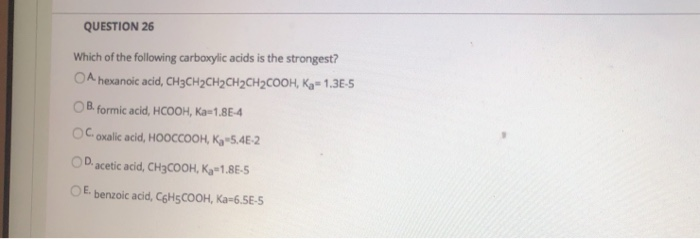QUESTION 26 Which of the following carboxylic acids is the strongest? A hexanoic acid, CH3CH2CH2CH2CH2COOH, K.-1.3E-5 B. formic acid, HCOOH, Ka-1.8E-4 Coxalic acid, HOOCCOOH, K 5.4E-2 D. acetic acid, CH3COOH, K,-1.86-5 E benzoic acid, C6H5COOH, Ka-6.55-5

• ### Given the following K values, which anion is the strongest base? Acid H 4.2 x 10...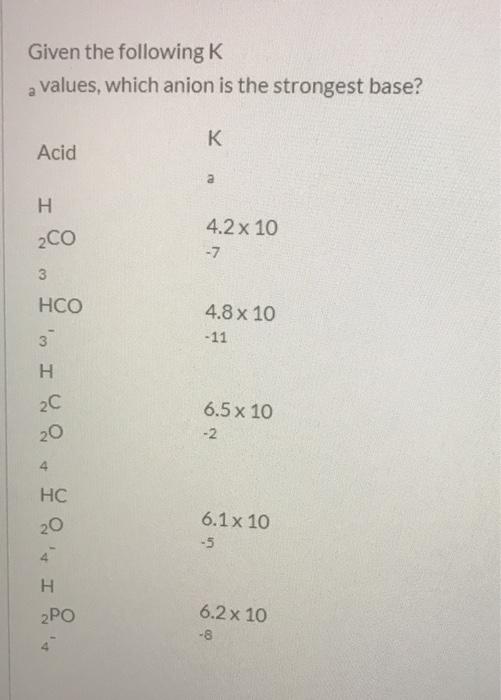Given the following K values, which anion is the strongest base? Acid H 4.2 x 10 4.8 x 10 - 11 6.5 x 10 6.1x 10 6.2 x 10 -8

• ### a) b) c) d) If the Kb of a weak acid is 2.9 10*, the K,...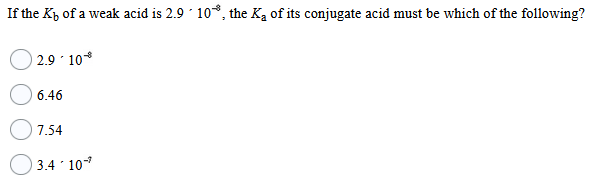a) b) c) d) If the Kb of a weak acid is 2.9 10*, the K, of its conjugate acid must be which of the following? 2.9.10 6.46 7.54 3.4 10- The hydronium ion concentration of a 0.50 mol/L solution of hydrocyanic acid, HCN(aq), is 1.4 x 10-5 mol/L. Use Weak Acid Info 16.1 to answer the following question. The Ka for hydrocyanic acid is: 3.9 x 10-10 1.4 x 10-5 5.0 x 10-2 6.2 x 10-10 An acetic acid-sodium...

• ### Given its acid dissociation constant (Ka) which of the following is the weakest acid? A-HClO2 (1.1...

Given its acid dissociation constant (Ka) which of the following is the weakest acid? A-HClO2 (1.1 × 10−2) B-HCOOH (1.8 × 10−4) C- HCN (4.9 × 10−10) D-HF (3.5 × 10−4)

• ### Dropdown is products or reactants. Given the following information: = formic acid hydrocyanic acid HCOOH HCN...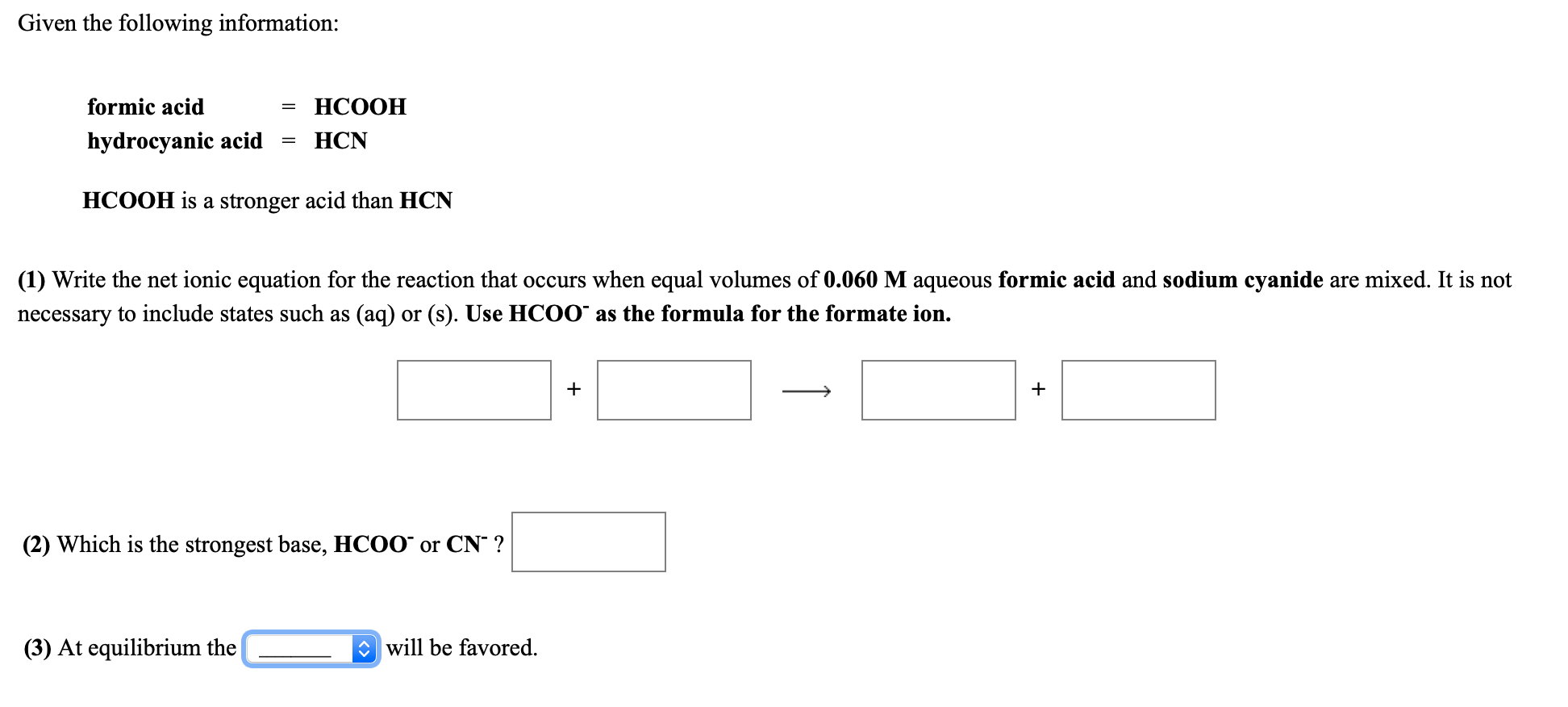Dropdown is products or reactants. Given the following information: = formic acid hydrocyanic acid HCOOH HCN HCOOH is a stronger acid than HCN (1) Write the net ionic equation for the reaction that occurs when equal volumes of 0.060 M aqueous formic acid and sodium cyanide are mixed. It is not necessary to include states such as (aq) or (s). Use HCOO as the formula for the formate ion. + + (2) Which is the strongest base, HCOO or CNº...

• ### which acid is the strongest ? Hydrogen sulfate ion HS04– (Ka = 1.2 x 10-2 Hydrofluoric...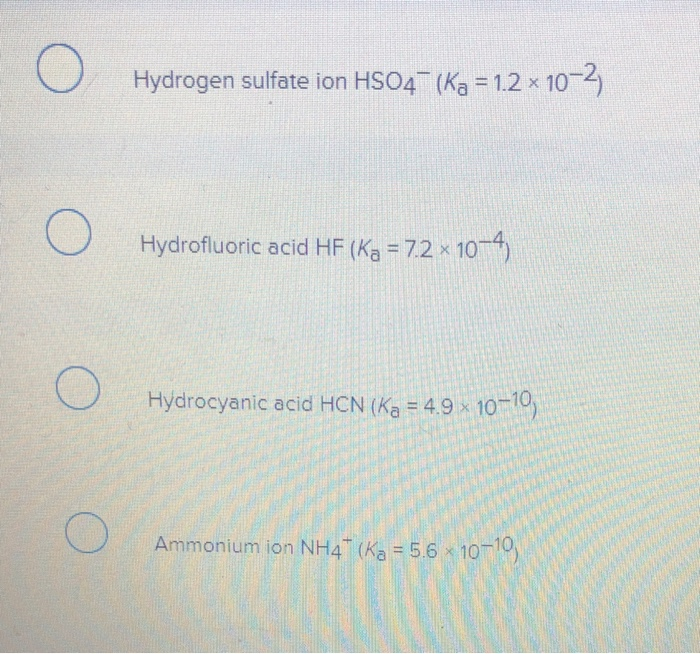which acid is the strongest ? Hydrogen sulfate ion HS04– (Ka = 1.2 x 10-2 Hydrofluoric acid HF (Ka = 7.2 x 10-4) Hydrocyanic acid HCN (Ka = 4.9 10–10, O Ammonium ion NH4" (ka = 5.6 10-10

• ### Which of the following acids is the strongest? The acid is followed by its Ka value....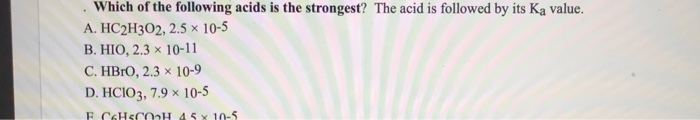Which of the following acids is the strongest? The acid is followed by its Ka value. A. HC2H302, 2.5 x 10-5 B. HIO, 2.3 x 10-11 C. HBrO, 2.3 x 10-9 D. HCIO3, 7.9 x 10-5 F CHSCH 45 x 10-5

Free Homework App

Scan Your Homework
to Get Instant Free Answers
Need Online Homework Help?

Get Answers For Free
Most questions answered within 3 hours.